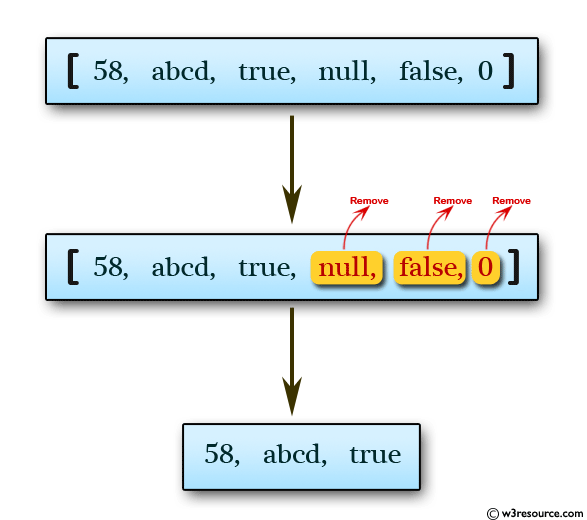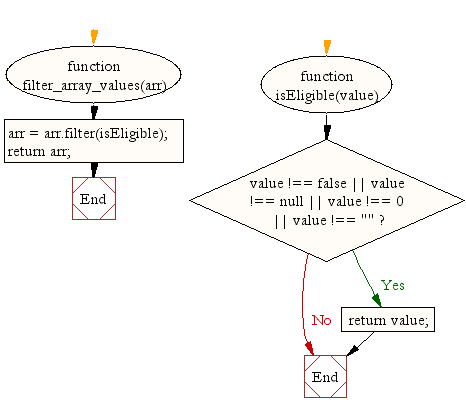# JavaScript: Filter false, null, 0 and blank values from an array

## JavaScript Array: Exercise-39 with Solution

Write a JavaScript function to filter false, null, 0 and blank values from an array.

Test Data :
console.log(filter_array_values([58, '', 'abcd', true, null, false, 0]));
[58, "abcd", true]

Pictorial Presentation:Sample Solution:

HTML Code:

``````<!DOCTYPE html>
<html>
<meta charset="utf-8">
<title>JavaScript function to filter false, null, 0 and blank values from an array</title>
<body>

</body>
</html>
```
```

JavaScript Code:

``````function filter_array_values(arr) {
arr = arr.filter(isEligible);
return arr;
}

function isEligible(value) {
if(value !== false || value !== null || value !== 0 || value !== "") {
return value;
}
}

console.log(filter_array_values([58, '', 'abcd', true, null, false, 0]));
```
```

Sample Output:

```[58,"abcd",true]
```

Flowchart:ES6 Version:

``````function filter_array_values(arr) {
arr = arr.filter(isEligible);
return arr;
}

function isEligible(value) {
if(value !== false || value !== null || value !== 0 || value !== "") {
return value;
}
}

console.log(filter_array_values([58, '', 'abcd', true, null, false, 0]));
``````

Live Demo:

See the Pen JavaScript - Filter false, null, 0 and blank values from an array-array-ex- 39 by w3resource (@w3resource) on CodePen.

Improve this sample solution and post your code through Disqus

What is the difficulty level of this exercise?

Test your Programming skills with w3resource's quiz.

﻿

```const sum = eval('10*10+5');